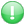Get premium membership and access revision papers, questions with answers as well as video lessons.

# Introduction To Business Statistics Question Paper

Course:Bachelor Of Commerce

Institution: Strathmore University question papers

Exam Year:2009

STRATHMORE UNIVERSITY
FACULTY OF COMMERCE
Bachelor of Commerce
END OF SEMESTER EXAMINATION
BCM 2105: INTRODUCTION TO BUSINESS STATISTICS
Venue: LT 2
Date: 14th October, 2009 Time: 2 Hours
INSTRUCTIONS: Answer Question ONE and any other TWO Questions

QUESTION ONE (30 marks)
a) Describe the measures of central tendency of a data set (4 marks)
b) Let x1, x2, ….,xn be n observations with corresponding frequencies f1, f2, …., fn’
Show that (i) the sum of deviations about the men is zero
(ii) Variance is not affected by change of scale (4 marks)
(c) In a statistics class of 30 students, the mean score on the mid-term test was 72. In
another class of 40 students, the mean score was 79. What is the mean for the two
classes combined? (4 marks)
e) A dairy farm company had two plots where dairy cows are kept. For one particular
day the milk yields from the two farms were as follows:
FARM 1 FARM 2
34 32 48 42 41 16 37 30 44 41
59 62 12 36 24 50 44 56 18 44
47 23 34 40 41 50 21 40 25 25
34 23 26 32 37 34 28 54 30 30
25 34 40 28 45 16 18 29 24 25 34
18 32 25 51 19 40 9 44 14 26 50
18 23 36 34 10 16 23 17 26 31 38
19 23 22 44 17 34 37
Required: Compare these data by constructing a back-to-back stem-and-leaf diagram
(6 marks)
f) Events A and B are such that P(A) = 0.3, P(B) = 0.4, P(AnB) = 0.1
Find (i) P(AnB’) Ii) P(A’nB’) (6 marks)
g) The values of random variable X and associated probabilities are given below:
x 2 3 4 5 6 7 8 9 10
p(x) 0.05 0.10 0.30 0.20 0.05 0.10 0.05 0.10 0.05
Calculate (i) E(X) (ii) E(5X + 3) (ii) Var(X) (iv) Standard deviation (6 marks)
2

QUESTION TWO (20 marks)
a) A finance analyst studied hundred companies and obtained the following data for
the year 2008:
Declared Dividends (K£’0000) Number of companies
0 – 8 10
8 – 16 20
16 – 24 16
24 – 32 19
32 – 40 15
40 – 48 10
48 – 56 6
56 – 64 4
Calculate (i) mean declared dividends (ii) Variance (iii) standard deviation of the
dividends (iv)median of number of the dividends
(12 marks)
b) At a certain University, students engage in sports in the following proportions:
Football: 40% of the total population
Hockey: 60% of total population
Both football and hockey: 30% of total population
If a student is chosen at random, calculate the probability that the student will play
(i) Football or hockey
(ii)Neither sport (6 marks)

QUESTION THREE (20 marks)
a) The data below shows yearly returns (millions) on equity of 50 companies in
2008.
165 141 163 153 130 158 119 187 185 209
177 147 166 154 159 178 187 139 180 143
160 185 153 168 189 173 127 179 163 182
171 146 174 149 126 156 155 174 154 150
210 162 138 117 198 164 125 142 182 218
Construct (i) a class frequency distribution with boundaries (ii) cumulative frequency
iii) draw a histogram (iii) draw a cumulative curve (ogive) (iv) estimate the
mode (v) estimate the median (14 marks)
b) A continuous random variable X has probability density function defined by
0 6
( )
0
kx x
f x
otherwise
?? = = =???
Find (i) value of k
(ii)P(1< x < 5)
(iii) Expected value of X
3
(iv) Variance of X (v) Standard deviation of X (8 marks)

QUESTION FOUR (20 marks)
(a) Define the terms (i) correlation coefficient (ii) coefficient of determination
(6 marks)
(b) Consider the following table that shows the amount of money used in sales and
Employee 1 2 3 4 5 6 7 8 9 10
Length of service
(years)
6 8 9 10 11 12 14 16 18 20
Annual
income(thousands)
14 17 15 18 16 22 26 25 30 34
Required: Find (i) correlation coefficient between length of service and the annual
income.
(ii) the coefficient of determination and interpret it
(iii) the regression equation of annual income on length of service
(iii) find the annual income when the length of service is 30 years
(14 marks)

QUESTION FIVE (20 marks)
(a) The usage rate per day in units of the A category inventory item over the last sixty
days is given below.
50, 100, 100, 150, 175, 200, 150, 50, 75, 150, 125, 50, 150, 140, 130,
200, 150, 140, 60, 125, 140, 130, 150, 150, 150, 140, 160, 150, 160, 200,
50, 125, 130, 150, 150, 125, 160, 150, 140, 140, 200, 100, 75, 100, 80,
120, 140, 150, 160, 150, 160, 175, 200, 140, 150, 160, 100, 100, 150.
The stores manger has requested you to:
(i) Construct a stem-and-leaf distribution
(ii) Construct a class frequency distribution of class size 5, 40 – 45, 45 – 50,
(iii) Compute mean, variance and standard deviation
. (12 marks)
(b) The hospital administrator at Nyalo Hospital requested a management consultant
to study the amount of time a patient must wait for being treated by the
emergency staff. The management consultant collected the following data during
a typical day:
Waiting times in (minutes)
10, 4, 5, 25, 35, 15, 5, 20, 12, 15, 10, 15, 13, 11, 14, 15, 35, 2, 10, 11,
13, 32
(i) Calculate the median and the quartiles
(ii) Calculate mean and standard deviation
(iii) Draw the box plot and hence identify the outliers
(iv) Calculate the coefficient of skewness and comment (8 marks)